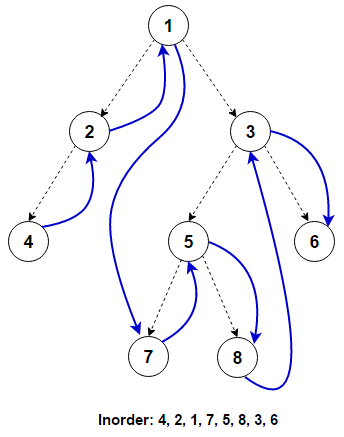# Inorder Tree Traversal – Iterative and RecursiveGiven a binary tree, write an iterative and recursive solution to traverse the tree using inorder traversal in C++, Java, and Python.

Unlike linked lists, one-dimensional arrays, and other linear data structures, which are traversed in linear order, trees can be traversed in multiple ways in depth–first order (preorder, inorder, and postorder) or breadth–first order (level order traversal). Beyond these basic traversals, various more complex or hybrid schemes are possible, such as depth-limited searches like iterative deepening depth–first search. In this post, inorder tree traversal is discussed in detail.

Traversing a tree involves iterating over all nodes in some manner. As the tree is not a linear data structure, there can be more than one possible next node from a given node, so some nodes must be deferred, i.e., stored in some way for later visiting. The traversal can be done iteratively where the deferred nodes are stored in the stack, or it can be done by recursion, where the deferred nodes are stored implicitly in the call stack.

For traversing a (non-empty) binary tree in an inorder fashion, we must do these three things for every node `n` starting from the tree’s root:

`(L)` Recursively traverse its left subtree. When this step is finished, we are back at `n` again.
`(N)` Process `n` itself.
`(R)` Recursively traverse its right subtree. When this step is finished, we are back at `n` again.

In normal inorder traversal, we visit the left subtree before the right subtree. If we visit the right subtree before visiting the left subtree, it is referred to as reverse inorder traversal.Practice this problem

## Recursive Implementation

As we can see, before processing any node, the left subtree is processed first, followed by the node, and the right subtree is processed at last. These operations can be defined recursively for each node. The recursive implementation is referred to as a Depth–first search (DFS), as the search tree is deepened as much as possible on each child before going to the next sibling.

Following is the C++, Java, and Python program that demonstrates it:

## Iterative Implementation

To convert the above recursive procedure into an iterative one, we need an explicit stack. Following is a simple stack-based iterative algorithm to perform inorder traversal:

iterativeInorder(node)

s —> empty stack
while (not s.isEmpty() or node != null)
if (node != null)
s.push(node)
node —> node.left
else
node —> s.pop()
visit(node)
node —> node.right

The algorithm can be implemented as follows in C++, Java, and Python:

## Python

The time complexity of the above solutions is O(n), where `n` is the total number of nodes in the binary tree. The space complexity of the program is O(n) as the space required is proportional to the height of the tree, which can be equal to the total number of nodes in the tree in worst-case for skewed trees.

References: https://en.wikipedia.org/wiki/Tree_traversal

Rate this post

Average rating 4.62/5. Vote count: 412

No votes so far! Be the first to rate this post.

We are sorry that this post was not useful for you!

Tell us how we can improve this post?